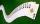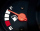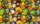# Fraction calculator

The calculator performs basic and advanced operations with fractions, expressions with fractions combined with integers, decimals, and mixed numbers. It also shows detailed step-by-step information about the fraction calculation procedure. Solve problems with two, three, or more fractions and numbers in one expression.

## Result:

### 13/4 + 2/3 = 29/12 = 2 5/12 ≅ 2.4166667

Spelled result in words is twenty-nine twelfths (or two and five twelfths).

### How do you solve fractions step by step?

1. Conversion a mixed number 1 3/4 to a improper fraction: 1 3/4 = 1 3/4 = 1 · 4 + 3/4 = 4 + 3/4 = 7/4

To find new numerator:
a) Multiply the whole number 1 by the denominator 4. Whole number 1 equally 1 * 4/4 = 4/4
b) Add the answer from previous step 4 to the numerator 3. New numerator is 4 + 3 = 7
c) Write a previous answer (new numerator 7) over the denominator 4.

One and three quarters is seven quarters
2. Add: 7/4 + 2/3 = 7 · 3/4 · 3 + 2 · 4/3 · 4 = 21/12 + 8/12 = 21 + 8/12 = 29/12
For adding, subtracting, and comparing fractions, it is suitable to adjust both fractions to a common (equal, identical) denominator. The common denominator you can calculate as the least common multiple of both denominators - LCM(4, 3) = 12. In practice, it is enough to find the common denominator (not necessarily the lowest) by multiplying the denominators: 4 × 3 = 12. In the next intermediate step, the fraction result cannot be further simplified by canceling.
In words - seven quarters plus two thirds = twenty-nine twelfths.

#### Rules for expressions with fractions:

Fractions - use the slash “/” between the numerator and denominator, i.e., for five-hundredths, enter 5/100. If you are using mixed numbers, be sure to leave a single space between the whole and fraction part.
The slash separates the numerator (number above a fraction line) and denominator (number below).

Mixed numerals (mixed fractions or mixed numbers) write as non-zero integer separated by one space and fraction i.e., 1 2/3 (having the same sign). An example of a negative mixed fraction: -5 1/2.
Because slash is both signs for fraction line and division, we recommended use colon (:) as the operator of division fractions i.e., 1/2 : 3.

Decimals (decimal numbers) enter with a decimal point . and they are automatically converted to fractions - i.e. 1.45.

The colon : and slash / is the symbol of division. Can be used to divide mixed numbers 1 2/3 : 4 3/8 or can be used for write complex fractions i.e. 1/2 : 1/3.
An asterisk * or × is the symbol for multiplication.
Plus + is addition, minus sign - is subtraction and ()[] is mathematical parentheses.
The exponentiation/power symbol is ^ - for example: (7/8-4/5)^2 = (7/8-4/5)2

#### Examples:

subtracting fractions: 2/3 - 1/2
multiplying fractions: 7/8 * 3/9
dividing Fractions: 1/2 : 3/4
exponentiation of fraction: 3/5^3
fractional exponents: 16 ^ 1/2
adding fractions and mixed numbers: 8/5 + 6 2/7
dividing integer and fraction: 5 ÷ 1/2
complex fractions: 5/8 : 2 2/3
decimal to fraction: 0.625
Fraction to Decimal: 1/4
Fraction to Percent: 1/8 %
comparing fractions: 1/4 2/3
multiplying a fraction by a whole number: 6 * 3/4
square root of a fraction: sqrt(1/16)
reducing or simplifying the fraction (simplification) - dividing the numerator and denominator of a fraction by the same non-zero number - equivalent fraction: 4/22
expression with brackets: 1/3 * (1/2 - 3 3/8)
compound fraction: 3/4 of 5/7
fractions multiple: 2/3 of 3/5
divide to find the quotient: 3/5 ÷ 2/3

The calculator follows well-known rules for order of operations. The most common mnemonics for remembering this order of operations are:
PEMDAS - Parentheses, Exponents, Multiplication, Division, Addition, Subtraction.
BEDMAS - Brackets, Exponents, Division, Multiplication, Addition, Subtraction
BODMAS - Brackets, Of or Order, Division, Multiplication, Addition, Subtraction.
GEMDAS - Grouping Symbols - brackets (){}, Exponents, Multiplication, Division, Addition, Subtraction.
Be careful, always do multiplication and division before addition and subtraction. Some operators (+ and -) and (* and /) has the same priority and then must evaluate from left to right.

## Fractions in word problems:

• ExpressionsLet k represent an unknown number, express the following expressions: 1. The sum of the number n and two 2. The quotient of the numbers n and nine 3. Twice the number n 4. The difference between nine and the number n 5. Nine less than the number n
• Fe metal sheetFor one product, 5/8 of the metal sheet are consumed, to the second 5/6 of remains. What part of the sheet metal is consumed for both products together?What is the sum of 2/3 and 3/10?Add this two mixed numbers: 1 5/6 + 2 2/11=Add two mixed fractions: 2 4/6 + 1 3/6
• Circular gardenAlice creates a circular vegetable garden. Tomatoes are planted in 1/3 of the circular garden, carrots are planted in 2/5 of the circular garden, and green peppers are planted in 1/10 of the circular garden. What fraction represents the remaining unplante
• Playing CardsKara has 2 times more cards than Dana, Dana has 4× less than Mary. Together they have 728 cards. How many cards has each of them?
• Math homeworkIt took Jose two-thirds of an hour to complete his math homework on Monday, three-fourths of an hour on Tuesday, any two- fifths of an hour on Wednesday. How many hours did it take Jose to complete his homework altogether?
• A textileA textile store sold a bolt of denim (what jeans are made out of). In one day, the following number of yards were purchased from the one bolt: 5 2/3, 7, 4 2/3, 8 5/8, 9 3/5, 10 ½, and 8. How many yards were sold?
• Monica’sMonica's lawnmower had 1/8 of a gallon of gasoline in the tank. Monica started mowing and used all of the gasoline. She put 6/10 of a gallon of gasoline in the tank. After she mowed, 1/4 of a gallon was left in the tank. What was the total amount of gasol
• MartinMartin is making a model of a Native American canoe. He has 5 1/2 feet of wood. He uses 2 3/4 feet for the hull and 1 1/4 feet for a paddle. How much wood does he have left? Martin has feet of wood left.
• CandiesThere are 150 candies in the bowl. Ivan took 7 thirtieths, Charles 6 fifteenths, and Thomas 3 tenths. How many sweets are left in the bowl?
• Infinite sum of areasAbove the height of the equilateral triangle ABC is constructed an equilateral triangle A1, B1, C1, of the height of the equilateral triangle built A2, B2, C2, and so on. The procedure is repeated continuously. What is the total sum of the areas of all tr